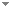Switch to: References

# A note on derivability conditions

Journal of Symbolic Logic 85 (3):1224-1253 (2020)

 On the Depth of Gödel’s Incompleteness Theorems.Yong Cheng - forthcoming - Philosophia Mathematica.ABSTRACT We use Gödel’s incompleteness theorems as a case study for investigating mathematical depth. We examine the philosophical question of what the depth of Gödel’s incompleteness theorems consists in. We focus on the methodological study of the depth of Gödel’s incompleteness theorems, and propose three criteria to account for the depth of the incompleteness theorems: influence, fruitfulness, and unity. Finally, we give some explanations for our account of the depth of Gödel’s incompleteness theorems. Direct download (2 more)     Export citationBookmarkVariants of Kreisel’s Conjecture on a New Notion of Provability.Paulo Guilherme Santos & Reinhard Kahle - 2021 - Bulletin of Symbolic Logic 27 (4):337-350.Kreisel’s conjecture is the statement: if, for all $n\in \mathbb {N}$, $\mathop {\text {PA}} \nolimits \vdash _{k \text { steps}} \varphi$, then $\mathop {\text {PA}} \nolimits \vdash \forall x.\varphi$. For a theory of arithmetic T, given a recursive function h, $T \vdash _{\leq h} \varphi$ holds if there is a proof of $\varphi$ in T whose code is at most $h$. This notion depends on the underlying coding. ${P}^h_T$ is a predicate for $\vdash _{\leq h}$ in (...) Direct download (2 more)     Export citationBookmarkOn the Invariance of Gödel’s Second Theorem with Regard to Numberings.Balthasar Grabmayr - forthcoming - Review of Symbolic Logic:1-34.The prevalent interpretation of Gödel’s Second Theorem states that a sufficiently adequate and consistent theory does not prove its consistency. It is however not entirely clear how to justify this informal reading, as the formulation of the underlying mathematical theorem depends on several arbitrary formalisation choices. In this paper I examine the theorem’s dependency regarding Gödel numberings. I introduce deviant numberings, yielding provability predicates satisfying Löb’s conditions, which result in provable consistency sentences. According to the main result of this paper (...) Direct download (2 more)     Export citationBookmark5 citations